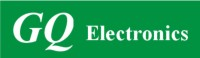.

GQ Electronics Technical Support Forum
Home | Profile | Register | Active Topics | Members | Search | FAQ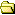All Forums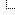GQ Electronics Forums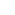3. GQ EMF EF Meter RF Spectrum Power Analyzer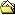Code for Output of data

Note: You must be registered in order to post a reply.

Screensize:
Format Mode: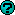Format: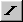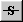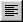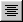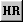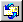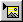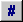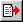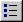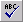Message:

* HTML is OFF
* Forum Code is ON
 Smilies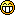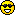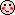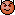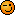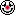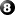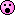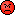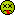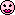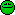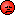Insert an Image File
Check here to include your profile signature.

 T O P I C    R E V I E W moeknows164 Posted - 06/22/2020 : 02:44:03 Is it possible to get a copy of the code how the data is sent to Excel 3   L A T E S T    R E P L I E S    (Newest First) EmfDev Posted - 06/23/2020 : 09:47:23 First you have to use the SPIR command to fetch the raw data from the unit. The end of the data can be regcognized by 0xFF 0xFF means no data in there.after fetching the data you have to decode.code is something like thistypedef union floatUnionT { float f; unsigned char uc;}floatUnionT;floatUnionT emf, ef, rf;for(unsigned long i = 0; i < END_OF_DATA; ){unsigned char byte1 = DATA[i++];unsigned char byte2 = DATA[i++];if((byte1 == 0x55) && (byte2 == 0xAA)) // time{ unsigned char time; for(int j = 0; j < 6; j++) time[j] = DATA[i++];}else if((byte1 == 0xAA) && (byte2 == 0x55)) // data{ unsigned char byte3 = DATA[i++]; unsigned char byte4 = DATA[i++]; unsigned int temp = ((unsigned int) byte3 << 8) | byte4; unsigned int emf_int = (temp >> 4) & 0x0FFF; unsigned char emf_dec = temp & 0x0F; emf.f = (emf_int + emf_dec/10); // emf.f is emf float ef.uc = DATA[i++]; ef.uc = DATA[i++]; ef.uc = DATA[i++]; ef.uc = DATA[i++]; rf.uc = DATA[i++]; rf.uc = DATA[i++]; rf.uc = DATA[i++]; rf.uc = DATA[i++]; float mwm2 = rf.f/100000; float mwcm2 = rf.f/1000000000; // Possible Source char sbuf; memset(sbuf,0,16); if(DATA[i] == 0x5A) { unsigned char s = DATA[i+1]; i+=2; if(s == 0) sprintf(sbuf, "---"); else if(s == 1) sprintf(sbuf, "Smart Meter"); else if(s == 2) sprintf(sbuf, "Cell Tower"); else if(s == 3) sprintf(sbuf, "Microwave"); else if(s == 4) sprintf(sbuf, "WiFi/Phone"); else if(s == 5) sprintf(sbuf, "Static"); else if(s == 6) sprintf(sbuf, "AC EF"); else if(s == 7) sprintf(sbuf, "Power Line"); else sprintf(sbuf, "Mixed"); } moeknows164 Posted - 06/22/2020 : 19:45:43 It is for the history data EmfDev Posted - 06/22/2020 : 09:53:00 Hi moeknows164, is it for the history data? or for the graph data?

 GQ Electronics Technical Support Forum © Copyright since 2011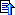Generated in 0.11 sec. Snitz's Forums 2000